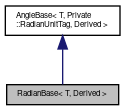MIRA
RadianBase< T, Derived > Class Template Reference

Base class for angle classes that represent angles using radians. More...

`#include <math/Angle.h>`

Inheritance diagram for RadianBase< T, Derived >:[legend]

## Public Member Functions

void reflect (Reflector &r)

void setValue (const T &value)
Set the angle value. The input value is mapped into the angle interval. More...

const T & value () const
Returns the raw angle value given in the native unit of the angle class. More...

operator T () const
Conversion function to native type (same as value() ) More...

void setSerializedValue (const T &value)
Sets the value in the unit that is used for serialization. More...

serializedValue () const
Returns the value in the unit that is used for serialization. More...

deg () const
Returns the value of the angle in degrees. More...

Returns the value of the angle in radian. More...

Derived operator- () const
Unary minus operator. More...

Derived operator+= (const AngleBase< T, OtherUnitTag, OtherDerived > &a)
Add other angle to this angle. More...

Derived & operator+= (const T &a)
Add float value to this angle. More...

Derived operator-= (const AngleBase< T, OtherUnitTag, OtherDerived > &a)
Subtract other angle from this angle. More...

Derived & operator-= (const T &a)
Subtract float value from this angle. More...

Derived & operator*= (const T &s)
Multiply this angle with scalar. More...

Derived & operator/= (const T &s)
Divide this angle by scalar. More...

smallestDifferenceValue (const Derived &other) const
Returns the signed difference angle between this angle and the specified other angle that has the smallest absolute value. More...

bool isInInterval (const Derived &min, const Derived &max) const
Returns true, if the angle is in the given interval [min,max]. More...

## Static Public Member Functions

static T turn ()
Returns the amount of a turn (full circle) that is equal to 360 deg. More...

static T convertToSerialized (T value)
Converts own representation to serialized representation. More...

static T convertFromSerialized (T value)
Converts serialized representation to own representation. More...

static T upper ()
Returns the upper limit of the defined angle interval. More...

static const char * unit ()
Returns the unit of this angle representation as string, e.g. More...

## Protected Attributes

mValue
the actual value More...

## Friends

class AngleBase< T, Private::RadianUnitTag, Derived >

## Detailed Description

### template<typename T, typename Derived> class mira::RadianBase< T, Derived >

Base class for angle classes that represent angles using radians.

## Member Function Documentation

 static T turn ( )
inlinestatic

Returns the amount of a turn (full circle) that is equal to 360 deg.

 static T convertToSerialized ( T value )
inlinestatic

Converts own representation to serialized representation.

 static T convertFromSerialized ( T value )
inlinestatic

Converts serialized representation to own representation.

 void reflect ( Reflector & r )
inlineinherited
 static T upper ( )
inlinestaticinherited

Returns the upper limit of the defined angle interval.

Note, that the upper limit does not belong to the interval itself, hence the interval is [lower,upper) .

 void setValue ( const T & value )
inlineinherited

Set the angle value. The input value is mapped into the angle interval.

 const T& value ( ) const
inlineinherited

Returns the raw angle value given in the native unit of the angle class.

 operator T ( ) const
inlineexplicitinherited

Conversion function to native type (same as value() )

Implicit conversion is intentionally disabled, in order to prevent potentially erroneous usage like

Angle a = 2;
T f = 5;
T r = a * f; // r is (T)Angle(2*5), which is NOT 2*5
 void setSerializedValue ( const T & value )
inlineinherited

Sets the value in the unit that is used for serialization.

The Angle and SignedAngle classes use radians for storing the value internal and degrees for serializing the value to simplify the usage for the human user. For the other classes Degree, SignedDegree, Radian and SignedRadian setValue() and setSerializedValue() are doing the same.

 T serializedValue ( ) const
inlineinherited

Returns the value in the unit that is used for serialization.

The Angle and SignedAngle classes use radians for storing the value internal and degrees for serializing the value to simplify the usage for the human user. For the other classes Degree, SignedDegree, Radian and SignedRadian value() and serializedValue() yield the same result.

 T deg ( ) const
inlineinherited

Returns the value of the angle in degrees.

inlineinherited

Returns the value of the angle in radian.

 Derived operator- ( ) const
inlineinherited

Unary minus operator.

 Derived operator+= ( const AngleBase< T, OtherUnitTag, OtherDerived > & a )
inlineinherited

Add other angle to this angle.

 Derived& operator+= ( const T & a )
inlineinherited

Add float value to this angle.

 Derived operator-= ( const AngleBase< T, OtherUnitTag, OtherDerived > & a )
inlineinherited

Subtract other angle from this angle.

 Derived& operator-= ( const T & a )
inlineinherited

Subtract float value from this angle.

 Derived& operator*= ( const T & s )
inlineinherited

Multiply this angle with scalar.

 Derived& operator/= ( const T & s )
inlineinherited

Divide this angle by scalar.

 T smallestDifferenceValue ( const Derived & other ) const
inlineinherited

Returns the signed difference angle between this angle and the specified other angle that has the smallest absolute value.

This method is similar to smallestDifference() but returns a floating point value instead of a signed angle class object.

 bool isInInterval ( const Derived & min, const Derived & max ) const
inlineinherited

Returns true, if the angle is in the given interval [min,max].

If max<min, both values will be swapped.

 static const char* unit ( )
inlinestaticinherited

Returns the unit of this angle representation as string, e.g.

## Friends And Related Function Documentation

 friend class AngleBase< T, Private::RadianUnitTag, Derived >
friend

## Member Data Documentation

 T mValue
protectedinherited

the actual value

The documentation for this class was generated from the following file: